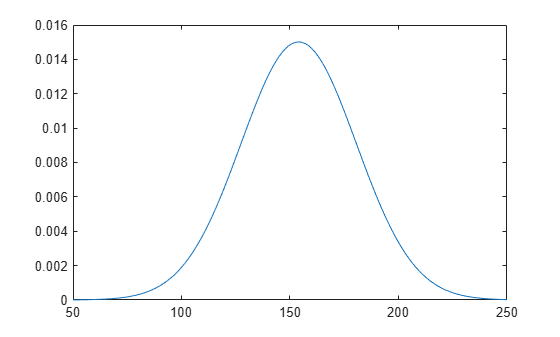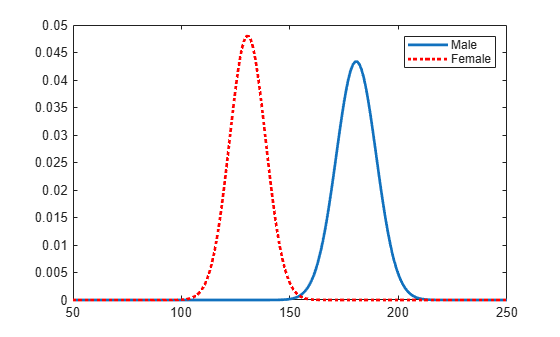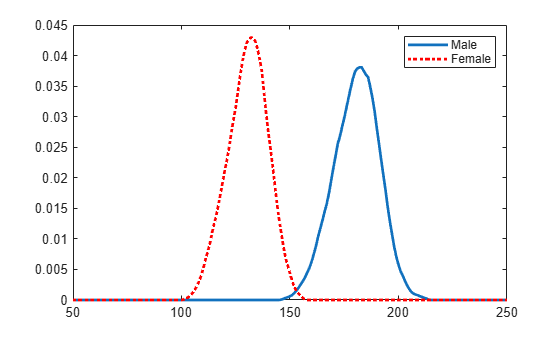Documentation

This is machine translation

Mouseover text to see original. Click the button below to return to the English version of the page.

fitdist

Fit probability distribution object to data

Syntax

``pd = fitdist(x,distname)``
``pd = fitdist(x,distname,Name,Value)``
``````[pdca,gn,gl] = fitdist(x,distname,'By',groupvar)``````
``````[pdca,gn,gl] = fitdist(x,distname,'By',groupvar,Name,Value)``````

Description

example

````pd = fitdist(x,distname)` creates a probability distribution object by fitting the distribution specified by `distname` to the data in column vector `x`.```

example

````pd = fitdist(x,distname,Name,Value)` creates the probability distribution object with additional options specified by one or more name-value pair arguments. For example, you can indicate censored data or specify control parameters for the iterative fitting algorithm.```

example

``````[pdca,gn,gl] = fitdist(x,distname,'By',groupvar)``` creates probability distribution objects by fitting the distribution specified by `distname` to the data in `x` based on the grouping variable `groupvar`. It returns a cell array of fitted probability distribution objects, `pdca`, a cell array of group labels, `gn`, and a cell array of grouping variable levels, `gl`.```

example

``````[pdca,gn,gl] = fitdist(x,distname,'By',groupvar,Name,Value)``` returns the above output arguments using additional options specified by one or more name-value pair arguments. For example, you can indicate censored data or specify control parameters for the iterative fitting algorithm.```

Examples

collapse all

Load the sample data. Create a vector containing the patients' weight data.

```load hospital x = hospital.Weight;```

Create a normal distribution object by fitting it to the data.

`pd = fitdist(x,'Normal')`
```pd = NormalDistribution Normal distribution mu = 154 [148.728, 159.272] sigma = 26.5714 [23.3299, 30.8674] ```

The intervals next to the parameter estimates are the 95% confidence intervals for the distribution parameters.

Plot the pdf of the distribution.

```x_values = 50:1:250; y = pdf(pd,x_values); plot(x_values,y,'LineWidth',2)```Load the sample data. Create a vector containing the patients' weight data.

```load hospital x = hospital.Weight;```

Create a kernel distribution object by fitting it to the data. Use the Epanechnikov kernel function.

`pd = fitdist(x,'Kernel','Kernel','epanechnikov')`
```pd = KernelDistribution Kernel = epanechnikov Bandwidth = 14.3792 Support = unbounded ```

Plot the pdf of the distribution.

```x_values = 50:1:250; y = pdf(pd,x_values); plot(x_values,y)```Load the sample data. Create a vector containing the patients' weight data.

```load hospital x = hospital.Weight;```

Create normal distribution objects by fitting them to the data, grouped by patient gender.

```gender = hospital.Sex; [pdca,gn,gl] = fitdist(x,'Normal','By',gender)```
```pdca = 1x2 cell array {1x1 prob.NormalDistribution} {1x1 prob.NormalDistribution} ```
```gn = 2x1 cell array {'Female'} {'Male' } ```
```gl = 2x1 cell array {'Female'} {'Male' } ```

The cell array `pdca` contains two probability distribution objects, one for each gender group. The cell array `gn` contains two group labels. The cell array `gl` contains two group levels.

View each distribution in the cell array `pdca` to compare the mean, `mu`, and the standard deviation, `sigma`, grouped by patient gender.

`female = pdca{1} % Distribution for females`
```female = NormalDistribution Normal distribution mu = 130.472 [128.183, 132.76] sigma = 8.30339 [6.96947, 10.2736] ```
`male = pdca{2} % Distribution for males`
```male = NormalDistribution Normal distribution mu = 180.532 [177.833, 183.231] sigma = 9.19322 [7.63933, 11.5466] ```

Compute the pdf of each distribution.

```x_values = 50:1:250; femalepdf = pdf(female,x_values); malepdf = pdf(male,x_values);```

Plot the pdfs for a visual comparison of weight distribution by gender.

```figure plot(x_values,femalepdf,'LineWidth',2) hold on plot(x_values,malepdf,'Color','r','LineStyle',':','LineWidth',2) legend(gn,'Location','NorthEast') hold off```Load the sample data. Create a vector containing the patients' weight data.

```load hospital x = hospital.Weight;```

Create kernel distribution objects by fitting them to the data, grouped by patient gender. Use a triangular kernel function.

```gender = hospital.Sex; [pdca,gn,gl] = fitdist(x,'Kernel','By',gender,'Kernel','triangle');```

View each distribution in the cell array `pdca` to see the kernel distributions for each gender.

`female = pdca{1} % Distribution for females`
```female = KernelDistribution Kernel = triangle Bandwidth = 4.25894 Support = unbounded ```
`male = pdca{2} % Distribution for males`
```male = KernelDistribution Kernel = triangle Bandwidth = 5.08961 Support = unbounded ```

Compute the pdf of each distribution.

```x_values = 50:1:250; femalepdf = pdf(female,x_values); malepdf = pdf(male,x_values);```

Plot the pdfs for a visual comparison of weight distribution by gender.

```figure plot(x_values,femalepdf,'LineWidth',2) hold on plot(x_values,malepdf,'Color','r','LineStyle',':','LineWidth',2) legend(gn,'Location','NorthEast') hold off```Input Arguments

collapse all

Input data, specified as a column vector. `fitdist` ignores `NaN` values in `x`. Additionally, any `NaN` values in the censoring vector or frequency vector cause `fitdist` to ignore the corresponding values in `x`.

Data Types: `double`

Distribution name, specified as one of the following character vectors or string scalars. The distribution specified by `distname` determines the type of the returned probability distribution object.

Distribution NameDescriptionDistribution Object
`'Beta'`Beta distribution`BetaDistribution`
`'Binomial'`Binomial distribution`BinomialDistribution`
`'BirnbaumSaunders'`Birnbaum-Saunders distribution`BirnbaumSaundersDistribution`
`'Burr'`Burr distribution`BurrDistribution`
`'Exponential'`Exponential distribution`ExponentialDistribution`
`'ExtremeValue'`Extreme Value distribution`ExtremeValueDistribution`
`'Gamma'`Gamma distribution`GammaDistribution`
`'GeneralizedExtremeValue'`Generalized Extreme Value distribution`GeneralizedExtremeValueDistribution`
`'GeneralizedPareto'`Generalized Pareto distribution`GeneralizedParetoDistribution`
`'HalfNormal'`Half-normal distribution`HalfNormalDistribution`
`'InverseGaussian'`Inverse Gaussian distribution`InverseGaussianDistribution`
`'Kernel'`Kernel distribution`KernelDistribution`
`'Logistic'`Logistic distribution`LogisticDistribution`
`'Loglogistic'`Loglogistic distribution`LoglogisticDistribution`
`'Lognormal'`Lognormal distribution`LognormalDistribution`
`'Nakagami'`Nakagami distribution`NakagamiDistribution`
`'NegativeBinomial'`Negative Binomial distribution`NegativeBinomialDistribution`
`'Normal'`Normal distribution`NormalDistribution`
`'Poisson'`Poisson distribution`PoissonDistribution`
`'Rayleigh'`Rayleigh distribution`RayleighDistribution`
`'Rician'`Rician distribution`RicianDistribution`
`'Stable'`Stable distribution`StableDistribution`
`'tLocationScale'`t Location-Scale distribution`tLocationScaleDistribution`
`'Weibull'`Weibull distribution`WeibullDistribution`

Grouping variable, specified as a categorical array, logical or numeric vector, character array, string array, or cell array of character vectors. Each unique value in a grouping variable defines a group.

For example, if `Gender` is a cell array of character vectors with values `'Male'` and `'Female'`, you can use `Gender` as a grouping variable to fit a distribution to your data by gender.

More than one grouping variable can be used by specifying a cell array of grouping variables. Observations are placed in the same group if they have common values of all specified grouping variables.

For example, if `Smoker` is a logical vector with values `0` for nonsmokers and `1` for smokers, then specifying the cell array `{Gender,Smoker}` divides observations into four groups: Male Smoker, Male Nonsmoker, Female Smoker, and Female Nonsmoker.

Example: `{Gender,Smoker}`

Data Types: `categorical` | `logical` | `single` | `double` | `char` | `string` | `cell`

Name-Value Pair Arguments

Specify optional comma-separated pairs of `Name,Value` arguments. `Name` is the argument name and `Value` is the corresponding value. `Name` must appear inside quotes. You can specify several name and value pair arguments in any order as `Name1,Value1,...,NameN,ValueN`.

Example: `fitdist(x,'Kernel','Kernel','triangle')` fits a kernel distribution object to the data in `x` using a triangular kernel function.

Logical flag for censored data, specified as the comma-separated pair consisting of `'Censoring'` and a vector of logical values that is the same size as input vector `x`. The value is `1` when the corresponding element in `x` is a right-censored observation and `0` when the corresponding element is an exact observation. The default is a vector of `0`s, indicating that all observations are exact.

`fitdist` ignores any `NaN` values in this censoring vector. Additionally, any `NaN` values in `x` or the frequency vector cause `fitdist` to ignore the corresponding values in the censoring vector.

This argument is valid only if `distname` is `'BirnbaumSaunders'`, `'Burr'`, `'Exponential'`, `'ExtremeValue'`, `'Gamma'`, `'InverseGaussian'`, `'Kernel'`, `'Logistic'`, `'Loglogistic'`, `'Lognormal'`, `'Nakagami'`, `'Normal'`, `'Rician'`, `'tLocationScale'`, or `'Weibull'`.

Data Types: `logical`

Observation frequency, specified as the comma-separated pair consisting of `'Frequency'` and a vector of nonnegative integer values that is the same size as input vector `x`. Each element of the frequency vector specifies the frequencies for the corresponding elements in `x`. The default is a vector of `1`s, indicating that each value in `x` only appears once.

`fitdist` ignores any `NaN` values in this frequency vector. Additionally, any `NaN` values in `x` or the censoring vector cause `fitdist` to ignore the corresponding values in the frequency vector.

Data Types: `single` | `double`

Control parameters for the iterative fitting algorithm, specified as the comma-separated pair consisting of `'Options'` and a structure you create using `statset`.

Data Types: `struct`

Number of trials for the binomial distribution, specified as the comma-separated pair consisting of `'NTrials'` and a positive integer value. You must specify `distname` as `'Binomial'` to use this option.

Data Types: `single` | `double`

Threshold parameter for the generalized Pareto distribution, specified as the comma-separated pair consisting of `'Theta'` and a scalar value. You must specify `distname` as `'GeneralizedPareto'` to use this option.

Data Types: `single` | `double`

Location parameter for the half-normal distribution, specified as the comma-separated pair consisting of `'mu'` and a scalar value. You must specify `distname` as `'HalfNormal'` to use this option.

Data Types: `single` | `double`

Kernel smoother type, specified as the comma-separated pair consisting of `'Kernel'` and one of the following:

• `'normal'`

• `'box'`

• `'triangle'`

• `'epanechnikov'`

You must specify `distname` as `'Kernel'` to use this option.

Kernel density support, specified as the comma-separated pair consisting of `'Support'` and `'unbounded'`, `'positive'`, or a two-element vector.

 `'unbounded'` Density can extend over the whole real line. `'positive'` Density is restricted to positive values.

Alternatively, you can specify a two-element vector giving finite lower and upper limits for the support of the density.

You must specify `distname` as `'Kernel'` to use this option.

Data Types: `single` | `double` | `char` | `string`

Bandwidth of the kernel smoothing window, specified as the comma-separated pair consisting of `'Width'` and a scalar value. The default value used by `fitdist` is optimal for estimating normal densities, but you might want to choose a smaller value to reveal features such as multiple modes. You must specify `distname` as `'Kernel'` to use this option.

Data Types: `single` | `double`

Output Arguments

collapse all

Probability distribution, returned as a probability distribution object. The distribution specified by `distname` determines the class type of the returned probability distribution object.

Probability distribution objects of the type specified by `distname`, returned as a cell array.

Group labels, returned as a cell array of character vectors.

Grouping variable levels, returned as a cell array of character vectors containing one column for each grouping variable.

Algorithms

The `fitdist` function fits most distributions using maximum likelihood estimation. Two exceptions are the normal and lognormal distributions with uncensored data.

• For the uncensored normal distribution, the estimated value of the sigma parameter is the square root of the unbiased estimate of the variance.

• For the uncensored lognormal distribution, the estimated value of the sigma parameter is the square root of the unbiased estimate of the variance of the log of the data.

Alternative Functionality

App

The Distribution Fitter app opens a graphical user interface for you to import data from the workspace and interactively fit a probability distribution to that data. You can then save the distribution to the workspace as a probability distribution object. Open the Distribution Fitter app using `distributionFitter`, or click Distribution Fitter on the Apps tab.

 Johnson, N. L., S. Kotz, and N. Balakrishnan. Continuous Univariate Distributions. Vol. 1, Hoboken, NJ: Wiley-Interscience, 1993.

 Johnson, N. L., S. Kotz, and N. Balakrishnan. Continuous Univariate Distributions. Vol. 2, Hoboken, NJ: Wiley-Interscience, 1994.

 Bowman, A. W., and A. Azzalini. Applied Smoothing Techniques for Data Analysis. New York: Oxford University Press, 1997.